Sunday 25th July 2021# Classroom Key Stage 2 / KS2 - Maths Activities & Resources

## Welcome to the Maths section of the Key Stage 2 classroom. Within KS2 Maths you will find online @school assessment tests that will help children to enhance their learning and revise the subject of Maths at Key Stage 2 level.

•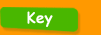•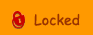••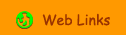•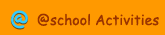•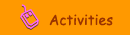•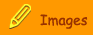###Mental Calculations

5 and a Bit - Interactive activity to add by partitioning into 5 and a bit.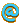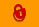Adding 9 - This activity teaches you a quick and easy way of adding 9 to any number.Adding Numbers to 20 - Interactive or printable activity to add numbers under 20Adding Two 2-Digit Numbers - Interactive activity to learn how to add two 2-digit numbers by partitioning into tens and units.Cross Sums - Interactive activity where you have to work out the different sums in a cross format.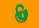Double Multiples of 10 - Interactive or printable activity to double multiples of 10Double Multiples of 10 - Activity 2 - Interactive or printable activity to double multiples of 10 up to 1000Double Multiples of 100 - Interactive or printable activity to double multiples of 100Double Multiples of 5 - Interactive or printable activity to double multiples of 5Double Multiples of 50 - Interactive or printable activity to double multiples of 50 to 500Double Numbers to 100 - Interactive or printable activity to double numbers to 100Double Numbers to 20 - Interactive or printable activity to double numbers to 20Double Numbers to 50 - Interactive or printable activity to double numbers to 50Doubling 2-Digit Numbers 1 - Interactive activity to learn how to double numbers with 2- digits.Doubling 3-Digit Numbers - Interactive activity to learn how to double numbers with 3- digits.Doubling Decimals - Interactive or printable activity to double decimal numbersHalve Multiples of 10 - Interactive or printable activity to halve multiples of 10Halve Numbers to 40 - Interactive or printable activity to halve numbers to 40Halving 2-Digit Numbers - Interactive activity to learn how to halve numbers with 2- digits.Halving 3-Digit Numbers - Interactive activity to learn how to halve numbers with 3 - digits.Halving Decimals - Interactive or printable activity to half decimal numbersMaking 100 - Interactive or printable activity to add multiples of 5 that total 100Making 100 part 2 - Interactive or printable activity to add pairs of numbers that total 100Making 1000 - Interactive or printable activity to add multiples of 100 that total 1000Making 1000 part 2 - Interactive or printable activity to add multiples of 50 that total 1000Prime number Calculator - Interactive calculator that tells you whether a number is prime or notSubtract a 2-Digit Number - Interactive activity to learn how to use partitioning to subtract 2-digit numbers.Subtracting Numbers to 20 - Interactive or printable activity to subtract numbers under 20